# Review — Zhu TMM’20: Generative Adversarial Network-Based Intra Prediction for Video Coding (HEVC/VVC Intra)

## Inpainting Using GAN for HEVC Intra Coding, Outperforms IPFCN.

In this story, Generative Adversarial Network-Based Intra Prediction for Video Coding, (Zhu TMM’20), by Chinese Academy of Sciences, City University of Hong Kong, City University of Hong Kong Shenzhen Institute, and Shenzhen University. In this paper:

• GAN is used to fill in the missing part by conditioning on the available reconstructed pixels for intra prediction in HEVC and VVC.
• This GAN inpainting is used as a intra coding prediction competing with the conventional intra prediction.

This is a paper in 2020 TMM where TMM has a high impact factor of 6.051. (Sik-Ho Tsang @ Medium)

# Outline

1. Brief Review of Conventional Intra Prediction
2. GAN Based Inpainting for Intra Prediction
3. Two Schemes for Applying GAN into Video Coding
4. Some Training Details
5. Experimental Results

# 1. Brief Review of Conventional Intra Prediction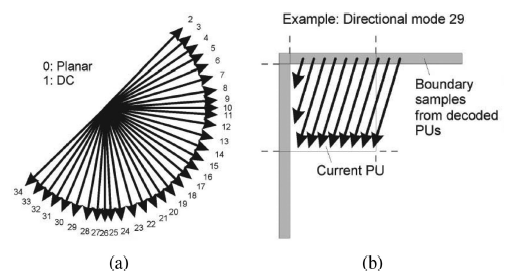(a) The 35 intra modes in HEVC. (b) Example of the angular mode 29.
• In HEVC intra mode, there are 35 intra predictions.
• Modes 0 and 1: They are planar and DC modes to predict smooth regions.
• Modes 2 to 34: They are angular predictions to extrapolate the boundary reference pixels.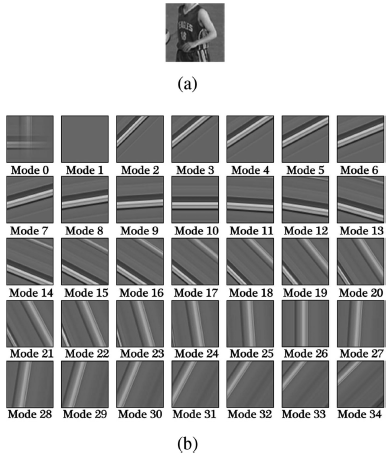(a) PU to be predicted (64 × 64). (b) Prediction results (modes 0–34).
• (a): It is the prediction unit (PU) to be predicted.
• (b): As seen, all 35 conventional intra predictions cannot predict the complex structure well.

Thus, in this paper, GAN is proposed for intra coding.

# 2. GAN Based Inpainting for Intra Prediction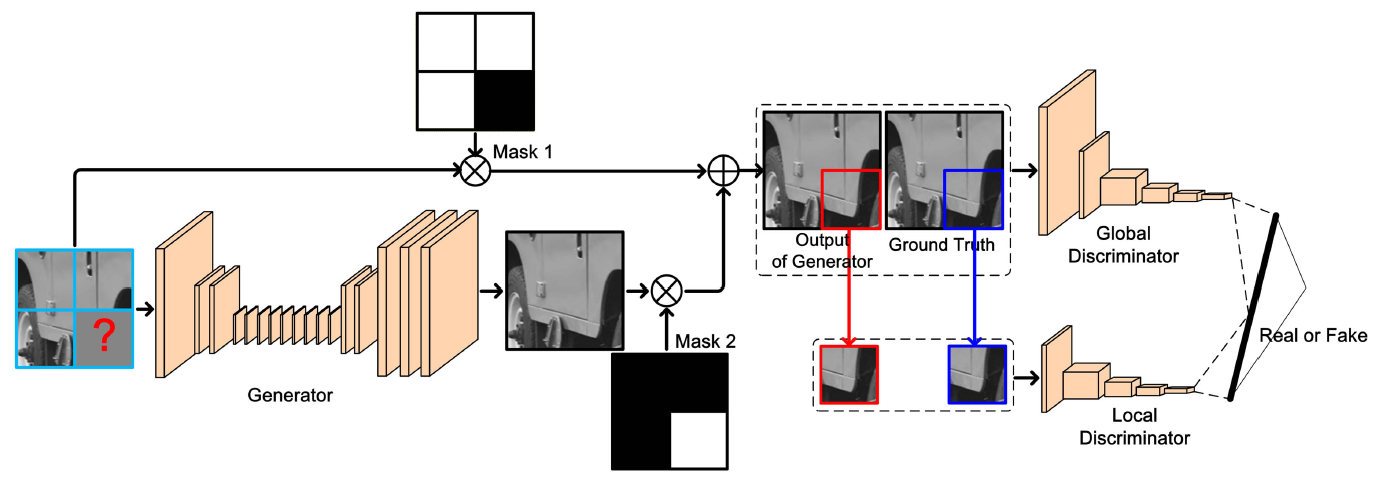Architecture of GAN based inpainting for intra prediction
• In this paper, the architecture is not the focus. The main novelty is how authors apply GAN as intra prediction to improve the coding efficiency.
• The architecture is mainly following the one in “Globally and locally consistent image completion”.
• The 128 × 128, with the missing part at the bottom right, and the reference pixels at the left, top-left and top, are input into the network.

• There are two masks with size of 128 × 128 applied to indicate the missing part. ‘⊗’ indicates pixelwise multiplication.
• In Mask 1, the values in the blocks of above-left, above and left are one, while the values in the block of bottom-right are zero.
• In Mask 2, the values are inverse to those in Mask 1.

## 2.2. Generator

• The generator G with 17 convolutional layers, which is used for predicting the missing part.

## 2.3. Discriminator

• The other is the discriminator D, which can be regarded as a binary classifier to identify whether the predicted missing part is real or fake.
• To improve the performance, there are two parts in the discriminator, i.e., local and global discriminators.
• For the local discriminator, there are 5 convolutional layers and 1 fully-connected layer, and the input is the predicted missing part.
• For the global discriminator, there are 6 convolutional layers and 1 fully-connected layer, and the input is the whole 128 × 128 image, where the missing part is the predicted and the other blocks are from the original input, as shown in the above figure.

## 2.4. 35 versions of prediction from GAN

• By filling 35 different colors at the missing part, 35 predictions from GAN are generated.
• The best one is the one with the lowest rate distortion cost.

# 3. Two Schemes for Applying GAN into Video Coding

• There are two schemes of GAN based inpainting for intra prediction.
• Scheme 1: One is 1 GAN model for 64 × 64 block. For 32 × 32, 16 × 16 and 8 × 8 blocks, the block prediction is copied from the 64 × 64 block prediction by the GAN according to the block size and position.
• Scheme 2: The other is 4 GAN models for 64 × 64, 32 × 32, 16 × 16 and 8 × 8 blocks.
• The below shows the advantages and disadvantages of both schemes:

Finally, Scheme 1 is adopted because of the benefits of simple implementation, less GAN models, less operations at the encoder side and easy adaptation to VVC.

# 4. Some Training Details

## 4.1. Training Dataset and Input

• The training dataset consists of 800 images with the resolution of 512 × 384 from an uncompressed color image database.
• They are encoded using QP 22.
• This sample and its corresponding ground truth without any coding distortion forms a training pair.
• Only luma component is extracted for training.
• The initial pixel values of missing part are randomly set for each sample during the training stage:
• where ⌊ ⋅ ⌋ is the function of floor round operation, X is randomly selected from {0, 1, 2, …, 34}, and k represents the bit depth.

## 4.2. Loss Functions

• The generator is trained for the first few epochs by the loss function of Mean Squared Error (MSE):
• where A1 and A2 are the local information, i.e. the missing parts of ground truth and predicted blocks.
• After a few epochs, the whole GAN network can be trained. For each training iteration, the generator and the discriminator will be repeatedly updated one by one. This is a min-max optimization problem:
• where B1 and B2 are the global information, i.e. the whole 128 × 128 image.
• α = 2500 to balance MSE loss and binary cross-entropy loss.

# 5. Experimental Results

• HM-16.17 is used. All intra configuration is used.
• The test sequences, which are different from the training data, are encoded with four QPs, including {22, 27, 32, 37}, under Common Test Conditions (CTC).

## 5.1. Intra Prediction Comparison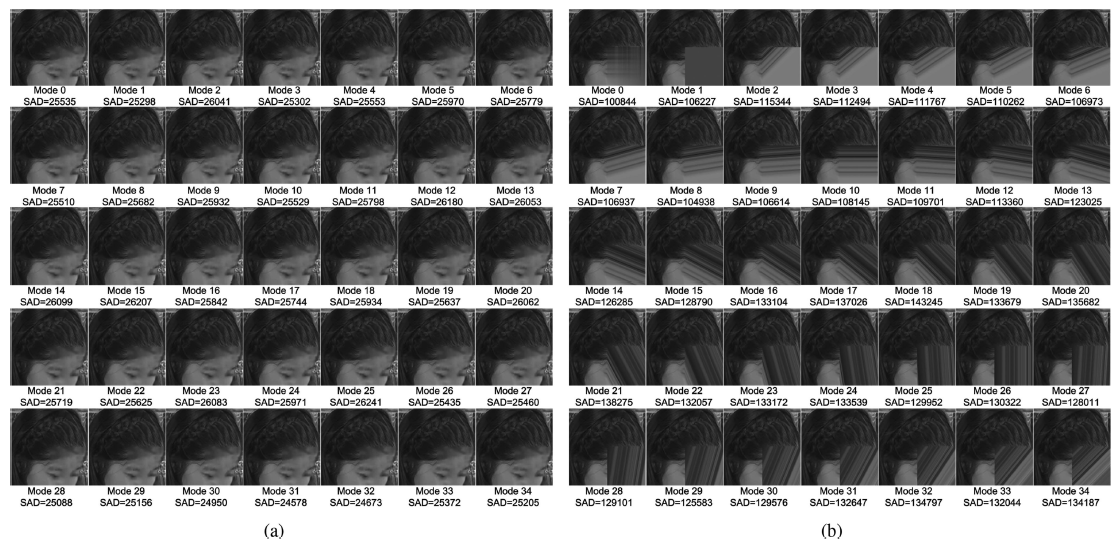Intra prediction comparison. (a) GAN based intra prediction. (b) Angular based intra prediction.
• The results from GAN based intra prediction are more consistent and have lower SADs.
• The best one is mode 31 with the minimum SAD value 24578.

## 5.2. Influence of Adversarial Term

• The results with adversarial term are more clear than those without adversarial term, and the MSE values are much smaller.
• The reason is that the local and global discriminators make the predicted pixel information consistent in the local and global.
• The inpainting for intra prediction with adversarial term can achieve more coding gains.

## 5.3. Comparison with SOTA Approaches

• For the proposed methods with 35 modes and 1 mode, they can achieve 6.6%, 7.5%, 7.5% and 6.2%, 7.2%, 7.5% on average bit rate reduction for luma and two chroma components, respectively, which is better than IPFCN .
• This is because the GAN based inpainting depends on the structural information of neighboring available blocks, such as the objects of face, and desk.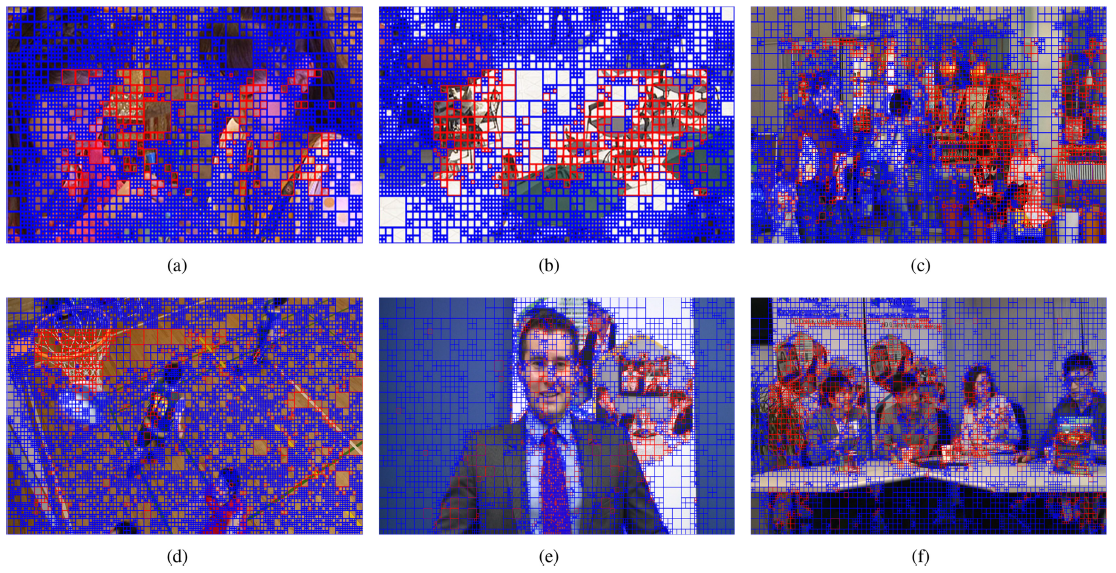Blue: Conventional Intra Prediction, Red: GAN-based intra prediction
• The blocks with GAN based intra prediction mainly locate at the texture area.

More structural information is in the neighboring blocks, higher probability the GAN based intra prediction will be selected.

• The high speed motion, and the characters, cannot be predicted by GAN.

## 5.4. Small QP Sets & Large QP Sets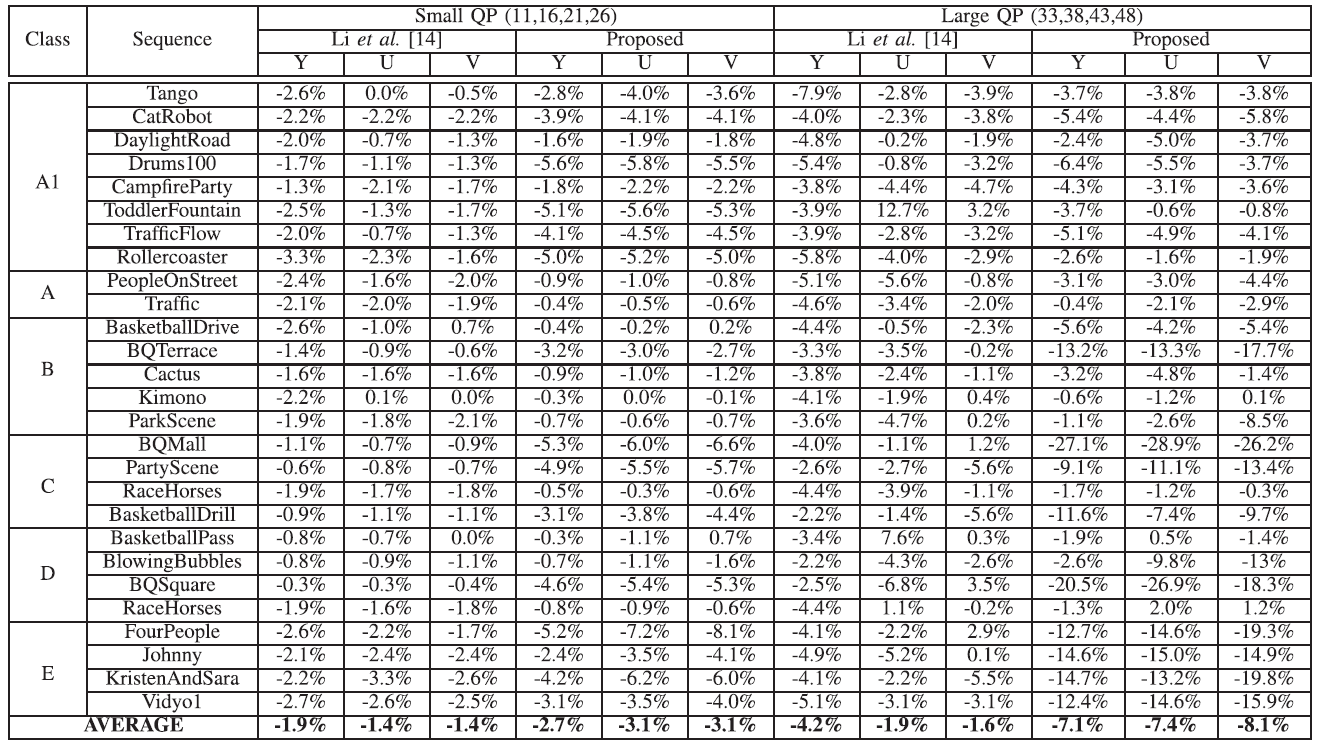BD-Rate (%) Using Different QP Ranges
• Using the same model but applying it into different QP ranges, there are also BD-rate reduction.

## 5.5. Adaptation to VVC

• The proposed method achieves 3.10%, 6.75% and 6.83% bit rate reduction in case of luma component under small, normal and large QP settings, respectively.

## 5.6. Computational Complexity Analysis

• Using CPU+GPU, under HM(VTM) the computational complexity of this proposed method is 7(2.5) and 160(257) times on average for encoding and decoding when compared with the original HM(VTM).
• Using CPU, the computational complexity of IPFCN and this proposed method is 86 and 149 times on average for encoding and 201 and 5264 times on average for decoding when compared with the original HM.

## Reference

[2020 TMM] [Zhu TMM’20]
Generative Adversarial Network-Based Intra Prediction for Video Coding

## Generative Adversarial Network (GAN)

Image Synthesis [GAN] [CGAN] [LAPGAN] [DCGAN] [Pix2Pix]
Super Resolution [SRGAN & SRResNet] [EnhanceNet] [ESRGAN]
Blur Detection [DMENet]
Camera Tampering Detection [Mantini’s VISAPP’19]
Video Coding
[VC-LAPGAN] [Zhu TMM’20]

## Codec Intra Prediction

JPEG [MS-ROI] [Baig JVICU’17]
HEVC
[Xu VCIP’17] [Song VCIP’17] [Li VCIP’17] [Puri EUSIPCO’17] [IPCNN] [IPFCN] [HybridNN, Li ICIP’18] [Liu MMM’18] [CNNAC] [Li TCSVT’18] [Spatial RNN] [PS-RNN] [AP-CNN] [MIP] [Wang VCIP’19] [IntraNN] [CNNAC TCSVT’19] [CNN-CR] [CNNMC Yokoyama ICCE’20] [PNNS] [CNNCP] [Zhu TMM’20]
VVC [CNNIF & CNNMC] [Brand PCS’19] [Bonnineau ICASSP’20] [Santamaria ICMEW’20] [Zhu TMM’20]

--

--